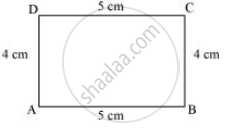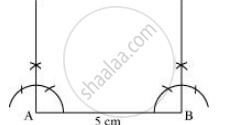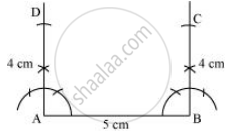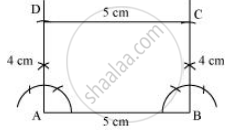# Draw the Following: a Rectangle with Adjacent Sides of Length 5 Cm and 4 Cm. - Mathematics

Draw the following:

A rectangle with adjacent sides of length 5 cm and 4 cm.

#### Solution

Opposite sides of a rectangle have their lengths of same measure and also, all the interior angles of a rectangle are of 90º measure. The given rectangle ABCD may be drawn as follows.

1) A rough sketch of this rectangle ABCD can be drawn as follows.2) Draw a line segment AB of 5 cm and an angle of 90º at point A and B.3) As vertex C and D are 4 cm away from vertex B and A respectively, cut line segments AD and BC, each of 4 cm, from these rays.]

4)  Join D to C.ABCD is the required rectangle.

Is there an error in this question or solution?
Chapter 4: Practical Geometry - Exercise 4.5 [Page 68]

#### APPEARS IN

NCERT Class 8 Maths
Chapter 4 Practical Geometry
Exercise 4.5 | Q 3 | Page 68

Share